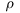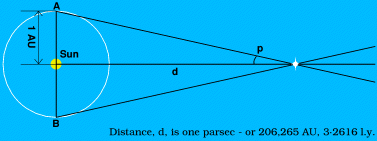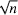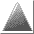# Glossary

[ SciRep | Graphing | Software | Test 1 | Test 2 | Test 3 | GraphDraw Reviewed ]
Anti-Aliasing
A remarkable Acorn invention to improve display of outline fonts, where the edges are made fuzzy, blending to the background. This process can also be applied to vector graphics, whereby lines and curves are smoothed, rather than having the classic computer staircase effect. The logo above is fully anti-aliased.

The following images, using the same font, illustrate the poor text display of a Windows™ PC, despite being in a 32-bit screen mode, when compared to the RISC OS display - which matches the printed document as closely as possible:

PC:Acorn:Astronomical Unit (symbol AU)
The Astronomical Unit used to be defined as the average distance from the Sun to the Earth. It is now defined as 1·496 × 108 km. Which is the same thing!
Arc Second (symbol " )
Angular measurement. 1/3600 of a degree.
Arc Minute (symbol ' )
Angular measurement. 1/60 of a degree.
bar (no symbol)
A unit of pressure, it is equivalent to 105 pascal (Pa). S.I. prefxes can be attached; e.g. mbar = millibar.
Bestfit or Best Line.
A straight line through a set of points on a graph. From this line, it is possible to predict the outcome of experiments outside the range of data; but to ever-increasing uncertainty.
CSV
Comma Separated Variable. The simplest form of file for holding scientific, or other, data. Data is listed in columns in a text file, each value being separated by a comma. Each new line represents a new set of data.
Cubic Spline
A curve fitted through, or close to, all the points on a graph. It takes points in small groups, and fits a simple cubic equation to fit these, then moves on to the next group. q.v. bestfit.
Degree (symbol °)
Angular measurement. A circle is divided uniformly into 360 degrees, so half a circle is 180°, a quarter 90°, etc.
Density (symbol- Greek letter, rho)
The amount of matter in a given volume of space, derived from mass/volume. Its basic unit is kg/m³, but g/cm³ is often used instead.
Ecliptic
The name given to the plane of the solar systm in which the planets approximately lie.
ESA
European Space Agency.
EXP
Exponential. Can have two meanings, but here we refer to exponential curves where the curve through data can be assymptotical to the axes, or other.
Frequency
The number of complete oscillations or cycles of a physical quantity in a unit time. It is the reciprocal of period, and the S.I. unit is the hertz (Hz).
hertz (no cap.; pl. hertz)
The S.I. unit of frequency, the same as cycles per second: 1 Hz = 1 s-1
Light Year (abbreviation ly)
The Light Year is defined as the distance travelled by light (or any other electro-magnetic wave) in a year in a vacuum. Since the speed is 3·00×108 m/s; and 1 year = 365 × 24 × 60 × 60 seconds = 3.15×107s; it follows that a light year is: 3·00×108 × 3.15×107 = 9.46×1015 m.
Log
Abbreviation and symbol for logarithm. The logarithm to the base a of x is written: loga x. The most common is a = 10, i.e. log10 x, or lg x.
Log-Log
A graphing method, whereby both the X and Y axes are logarithmic scales. See this in action!
LN
Natural Log - a logarithm, where a = 2.718 282... (a transcendental number), usually denoted loge x, or ln x.
mbar
See bar.
Origin
On a graph, the point where the x- and y- axes intersect, i.e. at co-ordinates (0,0).
Parabola
A curve of the form Y = u + vX + wX², where u, v and w are constants, X and Y the variables. The symbiosis between kinetic and gravitational energies in a simple harmonic system is an example of a parabolic relationship.
Parsec (symbol pc)A PARallax SECond. The diagram (right) shows how it is defined. The Earth's orbit is shown around the Sun. Over a period of 6 months, we move from A to B. A nearby star (white) will appear to change position against the background of more distant stars (not shown). The angular change in position (parallax) that the star undergoes is p. The parsec is defined as being the distance d for which p is 1 arc-second. No star is quite this close, but this unit is useful as if one can measure parallax, you can instantly find the distance in parsecs.
Pascal (symbol Pa)
The S.I. unit of pressure. 1 Pa = 1 Nm-2. Named after Blaise Pascal.
Period
The duration of one cycle or oscillation of a periodic phenomenon; i.e. the reciprocal of frequency. S.I. unit is the second.
Polynomial
A curve of the form y = a + bx + cx² + dx³ + ex4 + ..., where a, b, c, etc. are constants, x and y variables. Good graphing software typically can fit a polynomial to the sixth power to a set of data.
RADAR
RADAR stands for RAdio Detecting And Ranging.
Reciprocal
“One over x is the reciprocal of x.” i.e. 1/x, or x-1.
Retrograde
Literally meaning "going backwards", this can refer to the apparent backwards motion that planets appear to take in the sky when the Earth overtakes them. It can also refer to planetary moons orbiting in the opposite direction to the mother planet's rotation.
Scatter
A graph where the data is plotted as points only. Normally one would plot a curve through the points to show any trend in the data.
Semilog
A useful graphing method, whereby one of the X or Y axes has a logarithmic scale.
S.I.
Abbreviation for Système International (d'Unités)
SID
Software Independent Data format. An enhanced version of CSV, that includes information about graphs, electronic sensors, units, etc.
Spectroscopy
The study of light from stars or planets. A spectrum of light is produced by magnifying light from objects with a telescope, and separating it into colours with a prism or diffraction grating. The spectrum can reveal the chemical composition of the star, or constituents of a planet's atmosphere. More.
Square
“x × x = x squared.” i.e. x × x = x².
Square Rootor n½, where n is a number. The square root of n, multiplied by itself, is n.
TSV
Tab Separated Values. Just like a CSV file, but with variables separated by tabs (mainly used on Apple Macintosh computers).
watt, W
The unit of Power, that is the amount of energy used each second. One watt is equal to one joule per second. (`1W = 1J/s`)Page design by Andrew P. Harmsworth
Last updated Monday 5th January 2004